# Texas Go Math Grade 3 Lesson 9.3 Answer Key Multiply Multiples of 10 by 1-Digit Numbers

Refer to our Texas Go Math Grade 3 Answer Key Pdf to score good marks in the exams. Test yourself by practicing the problems from Texas Go Math Grade 3 Lesson 9.3 Answer Key Multiply Multiples of 10 by 1-Digit Numbers.

## Texas Go Math Grade 3 Lesson 9.3 Answer Key Multiply Multiples of 10 by 1-Digit Numbers

Essential Question
How can you model and record multiplying multiples of 10 by I-digit whole numbers?

Unlock the Problem
The community center offers 4 dance classes. If 30 students sign up for each class, how many students sign up for dance class altogether?

• How many equal groups are there? ___
• How many are in each group? ___

Activity
Use base-ten blocks to model 4 × 30.

Materialsbase-ten blocks

Step 1
Model 4 groups of 30.Step 2
Combine the tens. Regroup 12 tens as 1 hundred 2 tens.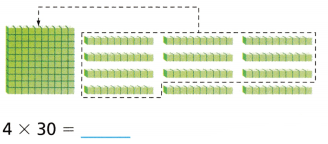Math Idea
If one factor is a multiple of 10, then the product will also be a multiple of 10.

Example
Use place value and regrouping. Find 7 × 40.Example
Use Mental Math and the Properties of Multiplication.

You can change the order or the grouping of the factors. The product is the same.

Math Idea
You can use mental math to multiply multiples of 10.Share and Show

Question 1.
Use the quick picture to find 5 × 40. ____
5 × 40 = __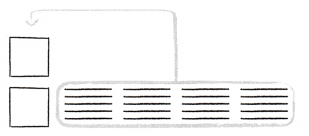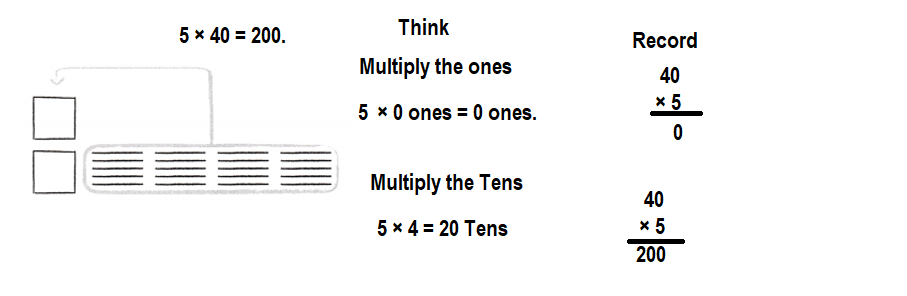Explanation:
Multiply the Ones.
5 × 0 = 0 ones.
Multiply the Tens.
5 × 4 = 20.
Re-grouping the ones and tens as hundreds and tens:
5 × 40 = 200.

Find the product.
Question 3.Explanation:
Multiply the Ones.
9 × 0 = 0 ones.
Multiply the Tens.
9 × 8 = 72.
Re-grouping the ones and tens as hundreds and tens:
9 × 80 = 720.

Question 4.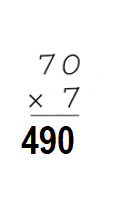Explanation:
Multiply the Ones.
7 × 0 = 0 ones.
Multiply the Tens.
7 × 7 = 49.
Re-grouping the ones and tens as hundreds and tens:
7 × 70 = 490.

Question 5.Explanation:
Multiply the Ones.
4 × 0 = 0 ones.
Multiply the Tens.
4 × 9 = 36.
Re-grouping the ones and tens as hundreds and tens:
4 × 90 = 360.

Question 6.Explanation:
Multiply the Ones.
8 × 0 = 0 ones.
Multiply the Tens.
8 × 6 = 48.
Re-grouping the ones and tens as hundreds and tens:
8 × 60 = 480.

Use mental math or properties to group the factors another way.
Then find the product.

Question 6.
4 × (2 × 30)
4 × (2 × 30) = 240.

Explanation:
4 × (2 × 30)
= (4 × 2 × 3) 10
= (8 × 3) 10
= 24 × 10
= 240.

Question 7.
(50 × 2) × 2
(50 × 2) × 2 = 200.

Explanation:
(50 × 2) × 2 = (10 × 5) × 2 × 2
= 10 (5 × 2 × 2)
= 10 (10 × 2)
= 10 × 20
= 200.

Question 8.
3 × (3 × 40)
3 × (3 × 40) = 360.

Explanation:
3 × (3 × 40) = 3 × 3 × (4 × 10)
= (3 × 3 × 4) × 10
= (9 × 4) 10
= 36 × 10
= 360.

Math Talk
Mathematical Processes
Explain how you can use place value to solve Exercise 5.

Algebra Find the unknown factor.

Question 9.
a × 80 = 480
a =___
a × 80 = 480
a = 8.

Explanation:
a × 80 = 480
=> a = 480 ÷ 80
=> a = 48 ÷ 8
=> a = 8.

Question 10.
b × 30 = 30
b = ___
b × 30 = 30
b = 1.

Explanation:
b × 30 = 30
=> b = 30 ÷ 30
=> b = 1.

Question 11.7 × ?? = 420.
?? = 60.

Explanation:
7 × ?? = 420
=> ?? = 420 ÷ 7
=> ?? = 60.

Question 12.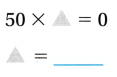50 × ?? = 0
?? = 0.

Explanation:
50 × ?? = 0
=> ?? = 0 ÷ 50
=> ?? = 0.

Problem Solving
Question 13.
Reasoning Multi-Step Ben writes the product of 7 and 50. Then he subtracts the least 3-digit multiple of 10 from the product. What is the difference?
Difference = 250.

Explanation:
Product of 7 and 50:
=> 7 × 50 = (7 × 10) + (7 × 10) + (7 × 10) + (7 × 10) + (7 × 10)
= 70 + 70 + 70 + 70 + 70
= 140 + 70 + 70 + 70
= 210 + 70 + 70
= 280 + 70
= 350.
Least 3-digit multiple of 10 = 100.
Difference:
Product of 7 and 50 – Least 3-digit multiple of 10
= 350 – 100
= 250.

Question 14.
H.O.T. Sense or Nonsense? Lori says that 8 is not a factor of 80 because 8 does not end in zero. Does Lori’s statement make sense? Explain.
Lori says that 8 is not a factor of 80 because 8 does not end in zero. Lori’s statement does make sense because 80 is a multiple of 8.

Explanation:
8 × ?? = 80.
=> ?? = 80 ÷ 8
=> ?? = 10.

Question 15.
Multi-Step Ava’s class bought 6 packages of balloons for a school celebration. Each package had 30 balloons. If 17 balloons were left over, how many balloons were used for the party?Number of balloons were used for the party = 163.

Explanation:
Number of packages of balloons for a school celebration Ava’s class bought = 6.
Number of balloons each package had = 30.
Number of balloons left over = 17.
Total number of balloons = Number of packages of balloons for a school celebration Ava’s class bought  × Number of balloons each package had
= 6 × 30
= (6 × 10) + (6 × 10) + (6 × 10)
= 60 + 60 + 60
= 120 + 60
= 180.
Number of balloons were used for the party = Total number of balloons – Number of balloons left over
= 180 – 17
= 163.

Question 16.
Analyze Frank has a 2-digit number on his baseball uniform. The number is a multiple of 10 and has 3 as one of its factors. What three numbers could Frank have on his uniform?
Three numbers could Frank have on his uniform:
=> 3 × 10 = 30.
=> 6 × 10 = 60.
=> 9 × 10 = 90.

Explanation:
Frank has a 2-digit number on his baseball uniform.
The number is a multiple of 10 and has 3 as one of its factors.
=> 3 × 10 = 30.
=> 6 × 10 = 60.
=> 9 × 10 = 90.

Question 17.
Write Math A landscaper planted 1 group of 30 trees in 9 different city parks. How many trees did he plant? Explain how you grouped your factors to solve the problem.
Number of trees he planted = 270.

Explanation:
Number of trees he planted = 30.
Number of different city parks he planted = 9.
Number of trees he planted = Number of trees he planted × Number of different city parks he planted
= 30 × 9
= (10 × 9) + (10 × 9) + (10 × 9)
= 90 + 90 + 90
= 180 + 90
= 270.

Daily Assessment Practice
Fill in the bubble for the correct answer choice.
Question 18.
A food carnival visited Morris Elementary School. During the show, 30 students each juggle 6 carrots. How many carrots do the Morris Elementary School students juggle?
(A) 180
(B) 36
(C) 60
(D) 6
Number of carrots the Morris Elementary School students juggle = 180.
(A) 180.

Explanation:
Number of students = 30.
Number of carrots each juggle = 6.
Number of carrots the Morris Elementary School students juggle = Number of students × Number of carrots each juggle
= 30 × 6
= 180.

Question 19.
Apply Asha bike jumps for 90 minutes every time she practices. She practices 4 days each week. How many minutes does Asha practice each week?
(A) 94
(B) 3,600
(C) 360
(D) 36
Number of minutes Asha practice each week = 360.
(C) 360.

Explanation:
Number of days she practiced each week = 4.
Time apply Asha bike jumps for every time she practices = 90 minutes.
Number of minutes Asha practice each week = Time apply Asha bike jumps for every time she practices × Number of days she practiced each week
= 90 × 4
= 10 (9 × 4)
= 10 × 36
= 360.

Question 20.
Multi-Step Vic makes ice pops in batches. He makes 2 batches of 20 cherry ice pops and 3 batches of 30 lime ice pops. How many ice pops does Vic make?
(A) 90
(B) 40
(C) 50
(D) 130
Number of ice pops Vic make = 130.
(D) 130.

Explanation:
Number of batches of cherry ice pops = 2.
Number of cherry ice pops = 20.
Total number of cherry ice pops = Number of batches of cherry ice pops × Number of cherry ice pops
= 2 × 20
= (2 × 10) + (2 × 10)
= 20 + 20
= 40.
Number of batches of lime ice pops = 3.
Number of lime ice pops = 30.
Total number of lime ice pops = Number of batches of lime ice pops × Number of lime ice pops
= 3 × 30
= (3 × 10) + (3 × 10) + (3 × 10)
= 30 + 30 + 30
= 60 + 30
= 90.
Number of ice pops Vic make = Total number of cherry ice pops + Total number of lime ice pops
= 40 + 90
= 10(4 + 9)
= 10 × 13
= 130.

Texas Test Prep

Question 21.
Wesley runs 10 miles each week for 2 weeks. Then he runs 20 miles each week for 3 weeks. How many miles does Wesley run in 5 weeks?
(A) 20 miles
(B) 80 miles
(C) 60 miles
(D) 30 miles
Number of miles Wesley run in 5 weeks = 80.
(B) 80 miles.

Explanation:
Number of miles Wesley runs each week = 10.
Number of weeks Wesley runs = 2.
Total number of weeks Wesley runs for 2 weeks = Number of miles Wesley runs each week × Number of weeks Wesley runs
= 10 × 2
= 20.
Number of miles Wesley runs each week = 20.
Number of weeks Wesley runs = 3.
Total number of weeks Wesley runs for 3 weeks = Number of miles Wesley runs each week × Number of weeks Wesley runs
= 20 × 3
= 60.
Number of miles Wesley run in 5 weeks = Total number of weeks Wesley runs for 2 weeks + Total number of weeks Wesley runs for 3 weeks
= 20 + 60
= 10(2 + 6)
= 10 × 8
= 80.

### Texas Go Math Grade 3 Lesson 9.3 Homework and Practice Answer Key

Find the product.

Question 1.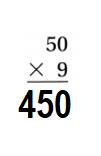Explanation:
Multiply the Ones.
9 × 0 = 0 ones.
Multiply the Tens.
9 × 5 = 45.
Re-grouping the ones and tens as hundreds and tens:
9 × 50 = 450.

Question 2.Explanation:
Multiply the Ones.
6 × 0 = 0 ones.
Multiply the Tens.
6 × 7 = 42.
Re-grouping the ones and tens as hundreds and tens:
6 × 70 = 420.

Question 3.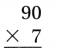Explanation:
Multiply the Ones.
7 × 0 = 0 ones.
Multiply the Tens.
7 × 9 = 63.
Re-grouping the ones and tens as hundreds and tens:
7 × 90 = 630.

Question 4.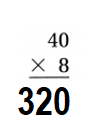Explanation:
Multiply the Ones.
8 × 0 = 0 ones.
Multiply the Tens.
8 × 4 = 32.
Re-grouping the ones and tens as hundreds and tens:
8 × 40 = 320.

Use mental math or properties to group the factors another way. Then find the product.

Question 5.
3 × (3 × 20)
3 × (3 × 20) = 180.

Explanation:
3 × (3 × 20)
= 3 × 3 × 2 × 10
= (3 × 3 × 2) 10
= (9 × 2) 10
= 18 × 10
= 180.

Question 6.
4 × (2 × 50)
4 × (2 × 50) = 400.

Explanation:
4 × (2 × 50)
= 4 × 2 × 5 × 10
= (4 × 2 × 5) 10
= (8 × 5) 10
= 40 × 10
= 400.

Question 7.
(60 × 2) × 4
(60 × 2) × 4 = 480.

Explanation:
(60 × 2) × 4
= 6 × 10 × 2 × 4
= (6 × 2 × 4) 10
= (12 × 4) 10
= 48 × 10
= 480.

Problem Solving

Question 8.
Rikki’s locker number is a two-digit number. The number is less than 70 and is an even multiple of 10. What three numbers could be Rikki’s locker number?
Three numbers could be Rikki’s locker number are
20, 40, 60.

Explanation:
Rikki’s locker number is a two-digit number.
The number is less than 70 and is an even multiple of 10.
=> The number is less than 70 = 10, 20, 30, 40, 50, 60.
=> Even multiple of 10 = 20, 40, 60.

Question 9.
Jerry brings 8 packages of paper plates to a picnic. Each package contains 20 plates. If 23 plates were left over, how many plates were used at the picnic?
Number of plates were used at the picnic = 137.

Explanation:
Number of packages of paper plates to a picnic Jerry brings = 8.
Number of plates each package contains = 20.
Number of plates were left over = 23.
Total number of plates = Number of packages of paper plates to a picnic Jerry brings × Number of plates each package contains
= 8 × 20
= (8 × 10) + (8 × 10)
= 80 + 80
= 160.
Number of plates were used at the picnic = Total number of plates – Number of plates were left over
= 160 – 23
= 137.

Question 10.
At the school play, there are 2 groups of seats. Each group of seats has 5 rows that hold 30 people. How many people can be seated at the play? Explain how you grouped your factors to solve the problem.
Number of people can be seated at the play = 300.

Explanation:
Number of groups of seats are at the school play = 2.
Number of seats of rows in each group = 5.
Number of people can seat in each row = 30.
Number of people can be seated at the play = Number of groups of seats are at the school play × Number of seats of rows in each group × Number of people can seat in each row
= 2 × 5 × 30
= (2 × 5 × 3) × 10
= (10 × 3) 10
= 30 × 10
= 300.

Texas test Prep

Lesson Check

Question 11.
Evelyn reads 40 pages of a book each night for 5 nights. How many pages does Evelyn read in all?
(A) 45
(B) 200
(C) 160
(D) 405
Number of pages Evelyn reads in all = 200.
(B) 200.

Explanation:
Number of pages of a book each night Evelyn reads = 40.
Number of nights Evelyn reads = 5.
Number of pages Evelyn reads in all = Number of pages of a book each night Evelyn reads × Number of nights Evelyn reads
= 40 × 5
= 10 × 4 × 5
= 10 × 20
= 200.

Question 12.
Lamar walks for 40 minutes every day. How many minutes does Lamar walk in one week?
(A) 280 minutes
(B) 210 minutes
(C) 200 minutes
(D) 320 minutes
Number of minutes Lamar walks in one week = 280.
(A) 280 minutes.

Explanation:
Time Lamar walks for every day = 40 minutes.
Number of days a week = 7.
Number of minutes Lamar walks in one week = Time Lamar walks for every day × Number of days a week
= 40 × 7
= 10 × 4 × 7
= 10 × 28
= 280.

Question 13.
Mr. Carmello drives 30 miles each way back and forth to work. If he works 4 days each week, how many miles does he drive in all?
(A) 120 miles
(B) 304 miles
(C) 240 miles
(D) 210 miles
Number of miles he drive in all = 120.
(A) 120 miles.

Explanation:
Number of miles each way back and forth to work Mr. Carmello drives = 30.
Number of days he works in a week = 4.
Number of miles he drive in all = Number of miles each way back and forth to work Mr. Carmello drives × Number of days he works in a week
= 30 × 4
= 10 × 3 × 4
= 10 × 12
= 120.

Question 14.
At the parade, there are 7 floats. Each float has 30 people riding on it. How many people ride on the floats at the parade?
(A) 308
(B) 420
(C) 210
(D) 320
Number of people ride on the floats at the parade = 210.
(C) 210.

Explanation:
Number of floats at the parade there are = 7.
Number of people riding on each float = 30.
Number of people ride on the floats at the parade = Number of floats at the parade there are × Number of people riding on each float
= 7 × 30
= 7 × 3 × 10
= 21 × 10
= 210.

Question 15.
Multi-Step Luisa has 2 rows of cabbage plants and 4 rows of lettuce plants. There are 30 plants in each row. How many plants does Luisa have?
(A) 200
(B) 180
(C) 120
(D) 240
Number of plants Luisa have = 120.
(C) 120.

Explanation:
Number of rows of cabbage plants Luisa has = 2.
Number of rows of lettuce plants Luisa has = 2.
Number of plants in each row = 30.
Number of plants Luisa have = Number of rows of cabbage plants Luisa has × Number of rows of lettuce plants Luisa has  × Number of plants in each row
= 2 × 2 × 30
= 2 × 2 × 3 × 10
= 4 × 3 × 10
= 12 × 10
= 120.

Question 16.
Multi-Step A store has 3 crates of sweet potatoes that each weigh 40 pounds. There are 4 crates of white potatoes that each weigh 50 pounds. How many pounds of potatoes are there?
(A) 280 pounds
(B) 360 pounds
(C) 420 pounds
(D) 320 pounds
Number of pounds of potatoes are there = 320.
(D) 320 pounds.

Explanation:
Number of crates of sweet potatoes a store has = 3.
Weight of each crates of sweet potatoes = 40 pounds.
Number of crates of white potatoes a store has = 4.
Weight of each crates of white potatoes = 50 pounds.
Number of pounds of potatoes are there = (Number of crates of sweet potatoes a store has × Weight of each crates of sweet potatoes) + (Number of crates of white potatoes a store has × Weight of each crates of white potatoes)
= (3 × 40) + (4 × 50)
= (3 × 4 × 10) + (4 × 5 × 10)
= (12 × 10) + ( 20 × 10)
= 120 + 200
= 320.

Scroll to Top